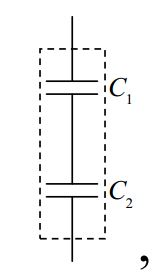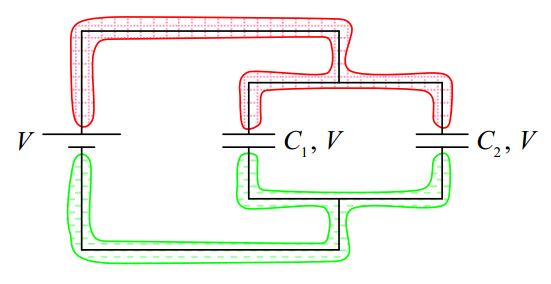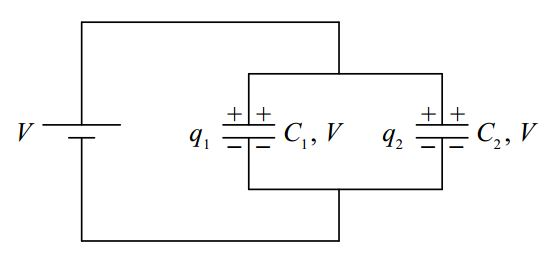$$\require{cancel}$$

# B14: Capacitors in Series & Parallel

The method of ever-simpler circuits that we used for circuits with more than one resistor can also be used for circuits having more than one capacitor. The idea is to replace a combination circuit element consisting of more than one capacitor with a single equivalent capacitor. The equivalent capacitor should be equivalent in the sense that, with the same potential across it, it will have the same charge as the combination circuit element.

# Capacitors in Series

Let’s start with a case in which the combination circuit element consists of two capacitors in series with each other:We consider the two capacitors to be a two-terminal combination circuit element:The voltage across the combination circuit element is clearly the EMF voltage V since, for both the seat of EMF and the combination circuit element, we’re talking about the potential difference between the same two conductors:The voltage across each individual capacitor is, however, not known.

But consider this: After that last wire is connected in the circuit, the charging process (which takes essentially no time at all) can be understood to proceed as follows (where, for ease of understanding, we describe things that occur simultaneously as if they occurred sequentially):

The seat of EMF pulls some positive charge from the bottom plate of the lower capacitor and pushes it onto the top plate of the upper capacitor.The key point about this movement of charge is that the amount of positive charge on the top plate of the upper capacitor is exactly equal to the amount of negative charge on the bottom plate of the lower capacitor (because that’s where the positive charge came from!)

Now, the positive charge on the upper plate of the top capacitor repels the positive charge (remember, every neutral object consists of huge amounts of both kinds of charge, and, in our positive-charge-carrier convention, the positive charges are free to move) on the bottom plate of the upper capacitor and that charge has a conducting path to the top plate of the lower capacitor, to which it (the positive charge) is attracted by the negative charge on the bottom plate of the lower capacitor.

The final result is that both capacitors have one and the same charge $$q$$:which in turn causes capacitor $$C_1$$ to have voltage $$V_1=\frac{q}{C_1}$$ and capacitor $$C_2$$ to have voltage $$V_2=\frac{q}{C_2}$$.By the loop rule,KVL 1

$V-V_1-V_2=0$

$V=V_1+V_2$

$V=\frac{q}{C_1}+\frac{q}{C_2}$

$V=q(\frac{1}{C_1}+\frac{1}{C_2})$

$q=\frac{1}{\frac{1}{C_1}+\frac{1}{C_2}}V$

So, what we’re saying is, that when you put a voltage $$V$$ across the two-terminal circuit elementan amount of charge $$q=\frac{1}{\frac{1}{C_1}+\frac{1}{C_2}}V$$ is moved from the bottom terminal of the combination circuit element, around the circuit, to the top terminal. Then charge stops moving. Recall that we defined the capacitance of a capacitor to be the ratio $$\frac{q}{V}$$ of the charge on the capacitor to the corresponding voltage across the capacitor. $$\frac{q}{V}$$ for our two-terminal combination circuit element is thus the equivalent capacitance of the two terminal circuit element. Solving $$q=\frac{1}{\frac{1}{C_1}+\frac{1}{C_2}}V$$ for the ratio $$\frac{q}{V}$$ yields $$\frac{q}{V}=\frac{1}{\frac{1}{C_1}+\frac{1}{C_2}}$$ so our equivalent capacitance for two capacitors in series is $$C_s=\frac{1}{\frac{1}{C_1}+\frac{1}{C_2}}$$By logical induction, we can extend this argument to cover any number of capacitors in series with each other, obtaining:

$C_s=\frac{1}{\frac{1}{C_1}+\frac{1}{C_2}+\frac{1}{C_3}+...}\label{14-1}$

As far as making things easy to remember, it’s just too bad the way things work out sometimes. This expression is mathematically identical to the expression for resistors in parallel. But, this expression is for capacitors in series.

# Capacitors in Parallel

Suppose we put a voltage $$V$$ across a combination circuit element consisting of a pair of capacitors in parallel with each other:It is clear from the diagram that the voltage across each capacitor is just the EMF $$V$$ since the voltage across every component in the circuit is the potential difference between the same two conductors.So what happens (almost instantaneously) when we make that final connection? Answer: The seat of EMF pulls charge off the bottom plates of the two capacitors and pushes it onto the top plates until the charge on $$C_1$$ is $$q_1 = C_1V$$ and the charge on $$C_2$$ is $$q_2 = C_2V$$.To do that, the seat of EMF has to move a total charge of

$q=q_1+q_2$

$q=C_1V+C_2V$

$q=(C_1+C_2)V$

Solving the last equation, $$q=(C_1+C_2)V$$, for the equivalent capacitance $$C_P$$, defined as $$q/V$$, yields:

$\frac{q}{V}=C_1+C_2$

$C_p=C_1+C_2$

In other words:So, the equivalent capacitance of capacitors in parallel is simply the sum of the individual capacitances. (This is the way resistors in series combine.) By means of inductive reasoning, the result can be extended to any number of capacitors, yielding:

$C_P=C_1+C_2+C_3+...\label{14-2}$

# Concluding Remarks

The facts that the voltage is the same for capacitors in parallel and the charge is the same for capacitors in series are important, but, if you look at these as two more things that you have to commit to memory then you are not going about your study of physics the right way. You need to be able to “see” that the charge on capacitors in series has to be the same because the charge on one capacitor comes from its (originally-neutral) neighbor. You need to be able to “see” that the voltage across capacitors in parallel has to be the same because, for each capacitor, the voltage is the potential difference between the same two conductors.